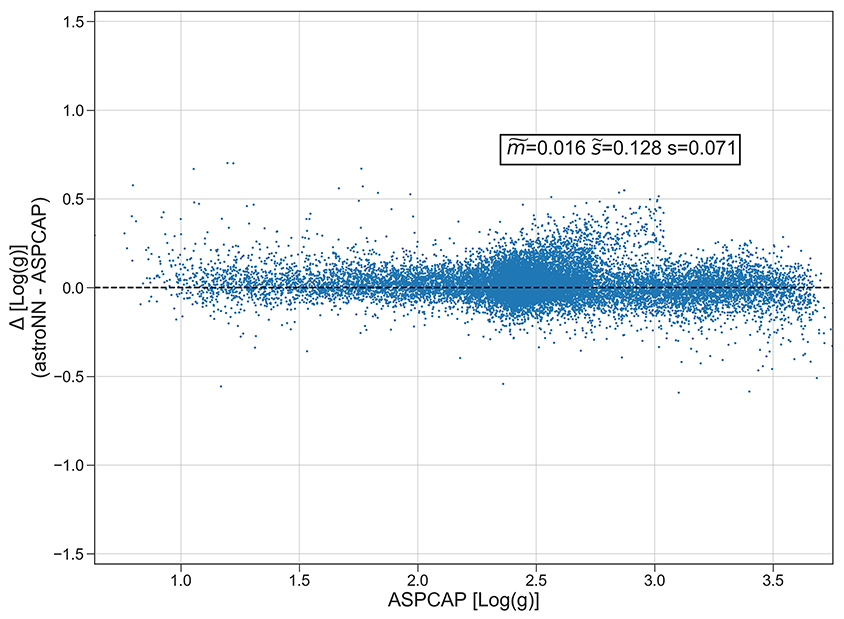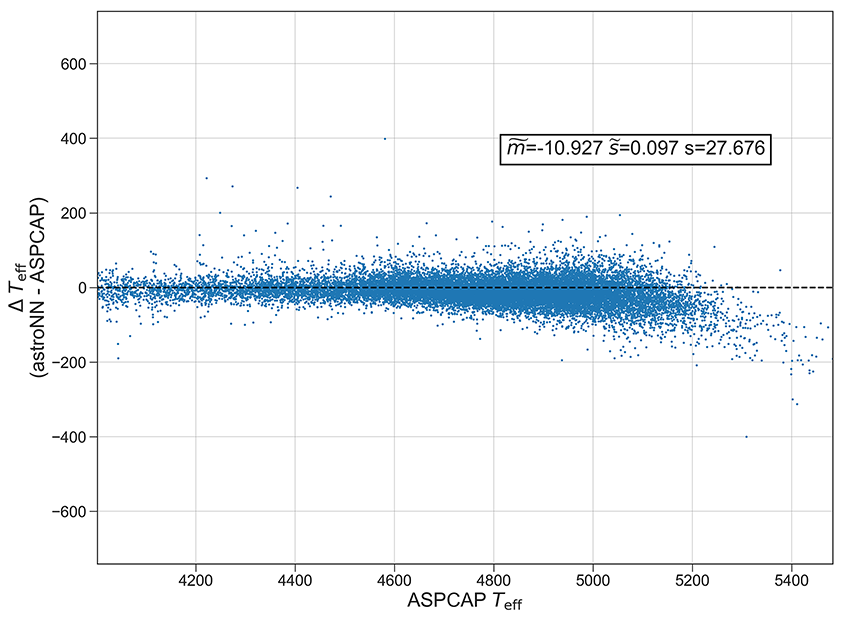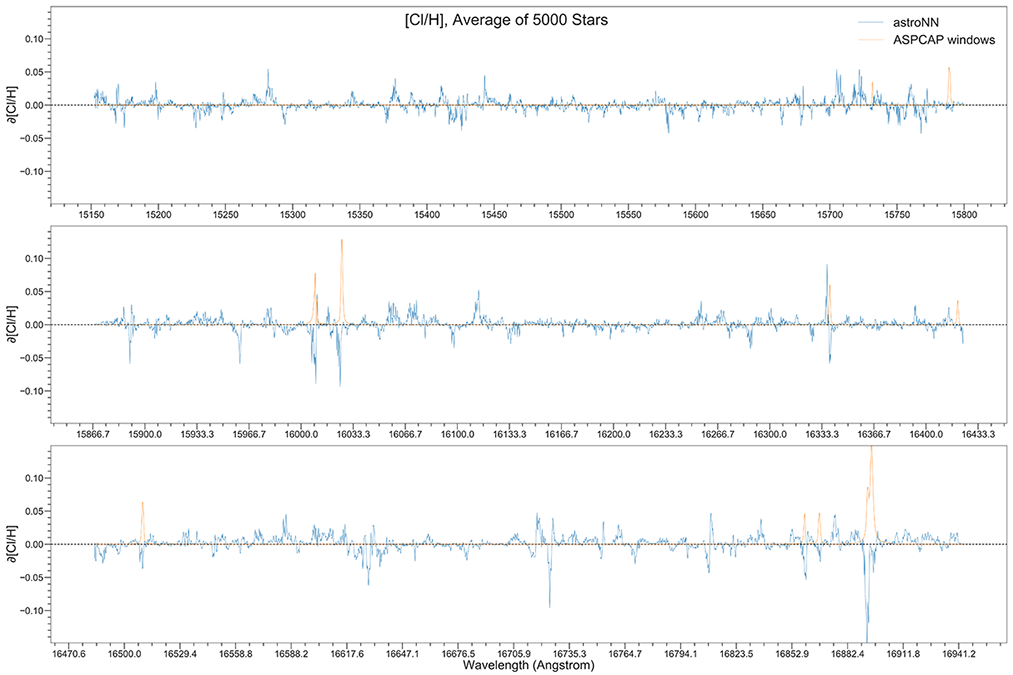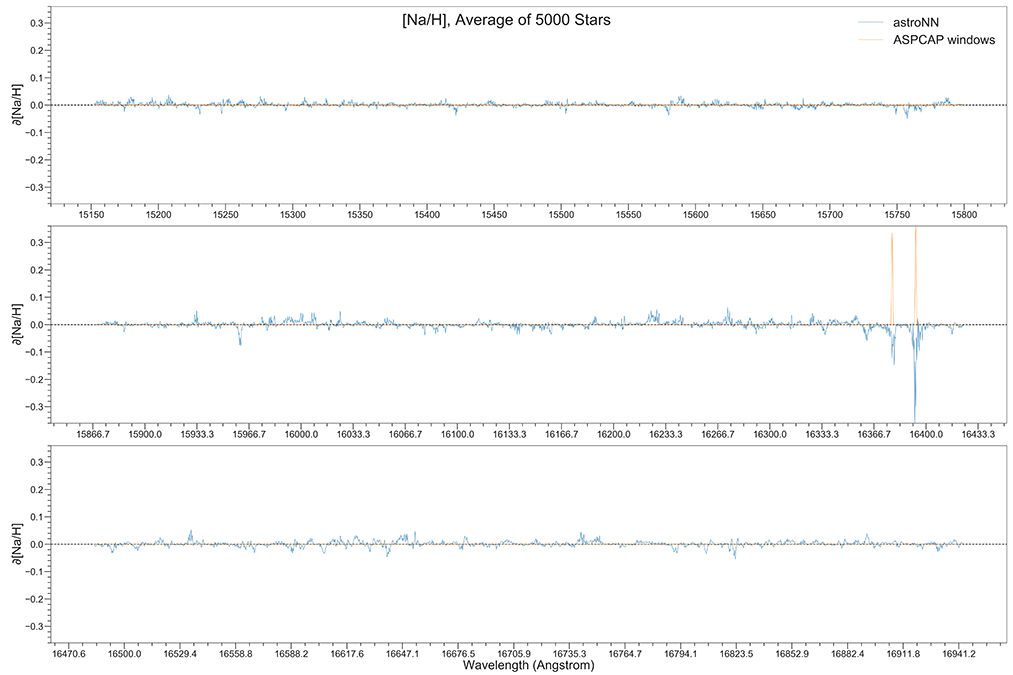# APOGEE Spectra with Convolutional Neural Net - astroNN.models.ApogeeCNN

class astroNN.models.apogee_models.ApogeeCNN(lr=0.005)[source]

Class for Convolutional Neural Network for stellar spectra analysis

History

2017-Dec-21 - Written - Henry Leung (University of Toronto)Although in theory you can feed any 1D data to astroNN neural networks. This tutorial will only focus on spectra analysis.

```from astroNN.models import ApogeeCNN

# Load the train data from dataset first, x_train is spectra and y_train will be ASPCAP labels

# And then create an instance of Convolutional Neural Network class
cnn_net = ApogeeCNN()

# You don't have to specify the task because its 'regression' by default. But if you are doing classification. you can set task='classification'

# Set max_epochs to 10 for a quick result. You should train more epochs normally
cnn_net.max_epochs = 10
cnn_net.train(x_train, y_train)
```

Here is a list of parameter you can set but you can also not set them to use default

```ApogeeCNN.batch_size = 64
ApogeeCNN.initializer = 'he_normal'
ApogeeCNN.activation = 'relu'
ApogeeCNN.num_filters = [2, 4]
ApogeeCNN.filter_len = 8
ApogeeCNN.pool_length = 4
ApogeeCNN.num_hidden = [196, 96]
ApogeeCNN.max_epochs = 250
ApogeeCNN.lr = 0.005
ApogeeCNN.reduce_lr_epsilon = 0.00005
ApogeeCNN.reduce_lr_min = 0.0000000001
ApogeeCNN.reduce_lr_patience = 10
ApogeeCNN.target = 'all'
ApogeeCNN.l2 = 1e-7
ApogeeCNN.input_norm_mode = 1
ApogeeCNN.labels_norm_mode = 2
```

Note

You can disable astroNN data normalization via `ApogeeCNN.input_norm_mode=0` as well as `ApogeeCNN.labels_norm_mode = 0` and do normalization yourself. But make sure you don’t normalize labels with `MAGIC_NUMBER` (missing labels).

After the training, you can use cnn_net in this case and call test method to test the neural network on test data. Or you can load the folder by

```from astroNN.models import load_folder

# Load the test data from dataset, x_test is spectra and y_test will be ASPCAP labels

pred = cnn_net.test(x_test)  # pred contains denormalized result aka. ASPCAP labels prediction in this case
```

Since astroNN.models.ApogeeCNN does not have uncertainty analysis feature.

You can calculate jacobian which represents the output derivative to the input and see where those output is sensitive to in inputs.

```# Calculate jacobian first
jacobian_array = cnn_net.jacobian(x_test, mean_output=True)
```

Note

You can access to Keras model method like model.predict via (in the above tutorial) cnn_net.keras_model (Example: cnn_net.keras_model.predict())

## Example Plots using aspcap_residue_plot## ASPCAP labels prediction using CNN vs The Cannon 2

Warning

Please refer to Bayesian Neural Network for the most updated result: http://astronn.readthedocs.io/en/latest/neuralnets/apogee_bcnn.html## Example Plots using jacobian